# Maharashtra Board 9th Class Maths Part 2 Problem Set 6 Solutions Chapter 6 Circle

Balbharti Maharashtra State Board Class 9 Maths Solutions covers the Problem Set 6 Geometry 9th Class Maths Part 2 Answers Solutions Chapter 6 Circle.

## Problem Set 6 Geometry 9th Std Maths Part 2 Answers Chapter 6 Circle

Question 1.
Choose correct alternative answer and fill in the blanks.

i. Radius of a circle is 10 cm and distance of a chord from the centre is 6 cm. Hence, the length of the chord is ____.
(A) 16 cm
(B) 8 cm
(C) 12 cm
(D) 32 cm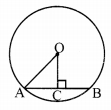∴ OA2 = AC2 + OC2
∴ 102 = AC2 + 62
∴ AC2 = 64
∴ AC = 8 cm
∴ AB = 2(AC)= 16 cm
(A) 16 cm

ii. The point of concurrence of all angle bisectors of a triangle is called the ____.
(A) centroid
(B) circumcentre
(C) incentre
(D) orthocentre
(C) incentre

iii. The circle which passes through all the vertices of a triangle is called ____.
(A) circumcircle
(B) incircle
(C) congruent circle
(D) concentric circle
(A) circumcircle

iv. Length of a chord of a circle is 24 cm. If distance of the chord from the centre is 5 cm, then the radius of that circle is ____.
(A) 12 cm
(B) 13 cm
(C) 14 cm
(D) 15 cmOA2 = AC2 + OC2
∴ OA2 = 122 + 52
∴ OA2 = 169
∴ OA = 13 cm
(B) 13 cm

v. The length of the longest chord of the circle with radius 2.9 cm is ____.
(A) 3.5 cm
(B) 7 cm
(C) 10 cm
(D) 5.8 cm
Longest chord of the circle = diameter = 2 x radius = 2 x 2.9 = 5.8 cm
(D) 5.8 cm

vi. Radius of a circle with centre O is 4 cm. If l(OP) = 4.2 cm, say where point P will lie ____.
(A) on the centre
(B) inside the circle
(C) outside the circle
(D) on the circle
∴Point P lies in the exterior of the circle.
(C) outside the circle

vii. The lengths of parallel chords which are on opposite sides of the centre of a circle are 6 cm and 8 cm. If radius of the circle is 5 cm, then the distance between these chords is _____.
(A) 2 cm
(B) 1 cm
(C) 8 cm
(D) 7 cmPQ = 8 cm, MN = 6 cm
∴ AQ = 4 cm, BN = 3 cm
∴ OQ2 = OA2 + AQ2
∴ 52 = OA2 + 42
∴ OA2 = 25 – 16 = 9
∴ OA = 3 cm
Also, ON2 = OB2 + BN2
∴ 52 = OB2 + 32
∴ OB = 4 cm
Now, AB = OA + OB = 3 + 4 = 7 cm

Question 2.
Construct incircle and circumcircle of an equilateral ADSP with side 7.5 cm. Measure the radii of both the circles and find the ratio of radius of circumcircle to the radius of incircle.
Solution: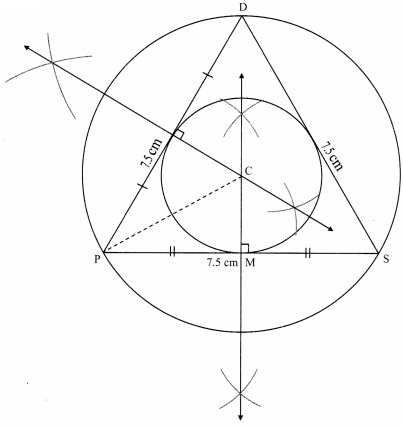Steps of construction:
i. Construct ∆DPS of the given measurement.
ii. Draw the perpendicular bisectors of side DP and side PS of the triangle.
iii. Name the point of intersection of the perpendicular bisectors as point C.
iv. With C as centre and CM as radius, draw a circle which touches all the three sides of the triangle.
v. With C as centre and CP as radius, draw a circle which passes through the three vertices of the triangle.

Radius of incircle = 2.2 cm and Radius of circumcircle = 4.4 cmQuestion 3.
Construct ∆NTS where NT = 5.7 cm. TS = 7.5 cm and ∠NTS = 110° and draw incircle and circumcircle of it.
Solution:Steps of construction:
For incircle:
i. Construct ∆NTS of the given measurement.
ii. Draw the bisectors of ∠T and ∠S. Let these bisectors intersect at point I.
iii. Draw a perpendicular IM on side TS. Point M is the foot of the perpendicular.
iv. With I as centre and IM as radius, draw a circle which touches all the three sides of the triangle.
For circumcircle:
i. Draw the perpendicular bisectors of side NT and side TS of the triangle.
ii. Name the point of intersection of the perpendicular bisectors as point C.
iii. Join seg CN
iv. With C as centre and CN as radius, draw a circle which passes through the three vertices of the triangle.

Question 4.
In the adjoining figure, C is the centre of the circle, seg QT is a diameter, CT = 13, CP = 5. Find the length of chord RS.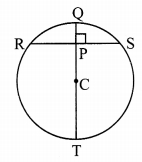Given: In a circle with centre C, QT is a diameter, CT = 13 units, CP = 5 units
To find: Length of chord RS
Construction: Join points R and C.
Solution:i. CR = CT= 13 units …..(i) [Radii of the same circle]
In ∆CPR, ∠CPR = 90°
∴ CR2 = CP2 + RP2 [Pythagoras theorem]
∴ 132 = 52 + RP2 [From (i)]
∴ 169 = 25 + RP2 [From (i)]
∴ RP2 = 169 – 25 = 144
∴ RP = $$\sqrt { 144 }$$ [Taking square root on both sides]
∴ RP = 12 cm ….(ii)

ii. Now, seg CP _L chord RS [Given]
∴ RP = $$\frac { 1 }{ 2 }$$ RS [Perpendicular drawn from the centre of the circle to the chord bisects the chord.]
∴ 12 = $$\frac { 1 }{ 2 }$$ RS [From (ii)]
∴ RS = 2 x 12 = 24
∴ The length of chord RS is 24 units.

Question 5.
In the adjoining figure, P is the centre of the circle. Chord AB and chord CD intersect on the diameter at the point E. If ∠AEP ≅ ∠DEP, then prove that AB = CD.Given: P is the centre of the circle.
Chord AB and chord CD intersect on the diameter at the point E. ∠AEP ≅ ∠DEP
To prove: AB = CD
Construction: Draw seg PM ⊥ chord AB, A-M-B
seg PN ⊥ chord CD, C-N-D
Proof:∠AEP ≅ ∠DEP [Given]
∴ Seg ES is the bisector of ∠AED.
PoInt P is on the bisector of ∠AED.
∴ PM = PN [Every point on the bisector of an angle is equidistant from the sides of the angle.]
∴ chord AB ≅ chord CD [Chords which are equidistant from the centre are congruent.]
∴ AB = CD [Length of congruent segments]

Question 6.
In the adjoining figure, CD is a diameter of the circle with centre O. Diameter CD is perpendicular to chord AB at point E. Show that ∆ABC is an isosceles triangle.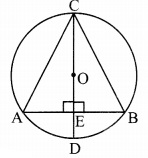Given: O is the centre of the circle.
diameter CD ⊥ chord AB, A-E-B
To prove: ∆ABC is an isosceles triangle.
Proof:
diameter CD ⊥ chord AB [Given]
∴ seg OE ⊥ chord AB [C-O-E, O-E-D]
∴ seg AE ≅ seg BE ……(i) [Perpendicular drawn from the centre of the circle to the chord bisects the chord]
In ∆CEA and ∆CEB,
∠CEA ≅ ∠CEB [Each is of 90°]
seg AE ≅ seg BE [From (i)]
seg CE ≅ seg CE [Common side]
∴ ∆CEA ≅ ∆CEB [SAS test]
∴ seg AC ≅ seg BC [c. s. c. t.]
∴ ∆ABC is an isosceles triangle.

Maharashtra Board Class 9 Maths Chapter 6 Circle Problem Set 6 Intext Questions and Activities

Question 1.
Every student in the group should do this activity. Draw a circle in your notebook. Draw any chord of that circle. Draw perpendicular to the chord through the centre of the circle. Measure the lengths of the two parts of the chord. Group leader should prepare a table as shown below and ask other students to write their observations in it. Write the property which you have observed. (Textbook pg. no. 77)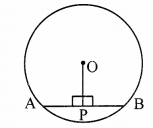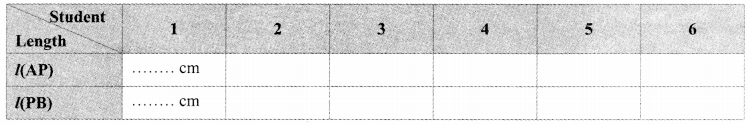On completing the above table, you will observe that the perpendicular drawn from the centre of a circle on its chord bisects the chord.

Question 2.
Every student from the group should do this activity. Draw a circle in your notebook. Draw a chord of the circle. Join the midpoint of the chord and centre of the circle. Measure the angles made by the segment with the chord.
Discuss about the measures of the angles with your friends. Which property do the observations suggest ? (Textbook pg. no. 77)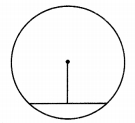The meausure of the angles made by the drawn segment with the chord is 90°. Thus, we can conclude that, the segment joining the centre of a circle and the midpoint of its chord is perpendicular to the chord.

Question 3.
Draw circles of convenient radii. Draw two equal chords in each circle. Draw perpendicular to each chord from the centre. Measure the distance of each chord from the centre. What do you observe? (Textbook pg. no. 79)
Congruent chords of a circle are equidistant from the centre.

Question 4.
Measure the lengths of the perpendiculars on chords in the following figures.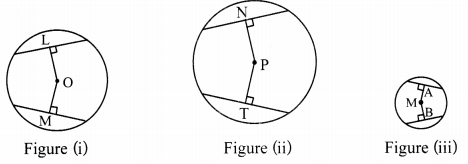Did you find OL = OM in fig (i), PN = PT in fig (ii) and MA = MB in fig (iii)?
Write the property which you have noticed from this activity. (Textbook pg. no. 80)